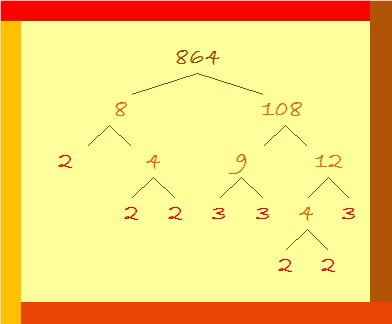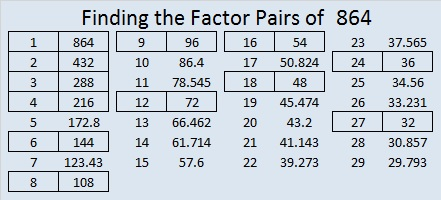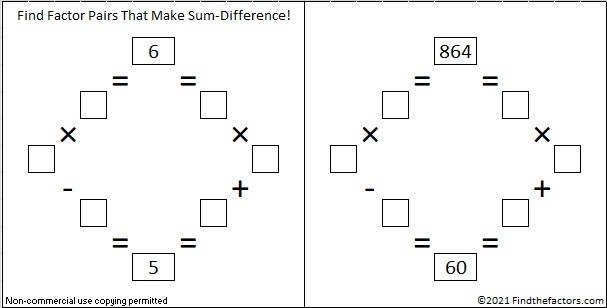# 864 Factor Trees

### Factor Trees for 864:

The prime factorization of 864 is 2⁵ × 3³. The sum of the exponents is 5 + 3 = 8. Since 8 is a power of 2,  a couple of 864’s factor trees are full and well-balanced:All of those prime factors lined up in numerical order. That didn’t happen for the next one, but it still makes a good looking tree, and all the prime factors are easy to find.Is it possible to make a factor tree for 864 that hardly looks like a tree and isn’t as easy to find all the prime factors? Yes, it is. Here’s an example:### Factors of 864:

• 864 is a composite number.
• Prime factorization: 864 = 2 × 2 × 2 × 2 × 2 × 3 × 3 × 3, which can be written 864 = 2⁵ × 3³
• The exponents in the prime factorization are 5 and 3. Adding one to each and multiplying we get (5 + 1)(3 + 1) = 6 × 4 = 24. Therefore 864 has exactly 24 factors.
• Factors of 864: 1, 2, 3, 4, 6, 8, 9, 12, 16, 18, 24, 27, 32, 36, 48, 54, 72, 96, 108, 144, 216, 288, 432, 864
• Factor pairs: 864 = 1 × 864, 2 × 432, 3 × 288, 4 × 216, 6 × 144, 8 × 108, 9 × 96, 12 × 72, 16 × 54, 18 × 48, 24 × 36, or 27 × 32
• Taking the factor pair with the largest square number factor, we get √864 = (√144)(√6) = 12√6 ≈ 29.3938769### Sum-Difference Puzzles:

6 has two factor pairs. One of those pairs adds up to 5, and the other one subtracts to 5. Put the factors in the appropriate boxes in the first puzzle.

864 has twelve factor pairs. One of the factor pairs adds up to ­60, and a different one subtracts to 60. If you can identify those factor pairs, then you can solve the second puzzle!### More Facts about the Number 864:

864 looked interesting to me in a few other bases:

• 4000 BASE 6 because 4(6³) = 864
• 600 BASE 12 because 6(12²) = 864
• RR BASE 31 (R is 27 base 10) because 27(31) + 27(1) = 27(32) = 864
• OO BASE 35 (O is 24 base 10) because 24(35) + 24(1) = 24(36) = 864
• O0 BASE 36 (Oh zero) because 24(36) + 0(1) = 864

864 is the sum of the 20 prime numbers from 7 to 83.

131 + 137 + 139 + 149 + 151 + 157 = 864; that’s six consecutive primes.

431 + 433 = 864; that’s the sum of twin primes.

864 is in this cool pattern: